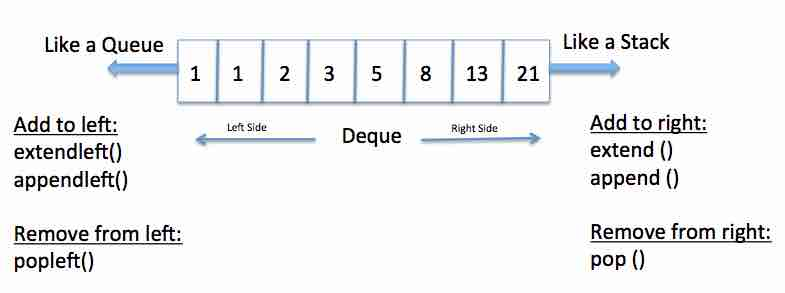# Deque In Python

## Overview

• deque is a container class in Python which can hold a collection of python objects.
• A deque is a double-ended queue on which elements can be added or removed from either side - that is on left end or right end, head or tail.
• A deque is like both a stack and queue.
• Adding an element or removing an element at either side of a deque instance takes constant time O(1).## Example:

 import collections   # create an empty deque d1 = collections.deque()   # add few elements to the right side of the deque d1.append(10) d1.append(10.1) d1.append("abc")   print("Contents of the deque") print(d1)

## Output:

 Contents of the deque deque([10, 10.1, 'abc'])

## Example2-Creation of a deque from an iterable object:

 import collections   # A tuple of prime numbers primeNumbers = (3,5,7)   #create a deque from an already existing iterable object - in this case a tuple primeDeque = collections.deque(primeNumbers) print("Initial deque:") print(primeDeque) primeDeque.append(11) primeDeque.append(13) primeDeque.append(13) primeDeque.appendleft(2) print("Deck after append and appendleft:") print(primeDeque)

## Output:

 Initial deque: deque([3, 5, 7]) Deck after append and appendleft: deque([2, 3, 5, 7, 11, 13, 13])

A python deque instance can be initialized using a python iterable object as well. Elements can be added to and removed from the deque subsequently from either side of the deque.

## Example3-Creation of a deque from an iterable object:

 import collections   # create a fixed size deque dequeInstance = collections.deque(maxlen=5)   # add 5 elements to the right side dequeInstance.append(10) dequeInstance.append(20) dequeInstance.append(30) dequeInstance.append(40) dequeInstance.append(50)   print(dequeInstance)   # add 1 element to the right side dequeInstance.append(60) print(dequeInstance)   # add 1 element to the left side dequeInstance.appendleft(5) print(dequeInstance)

## Output:

 deque([10, 20, 30, 40, 50], maxlen=5) deque([20, 30, 40, 50, 60], maxlen=5) deque([5, 20, 30, 40, 50], maxlen=5)

When the deque is specified of a maximum length using maxlen parameter and the deque has reached the maximum length, if an element is added to the left side one element is removed from the right side; when an element is added to the right side one element is removed from the left side.

## deque methods:

 append(elem) append() method adds one element to the right side of a deque instance, as specified the elem parameter. appendleft(elem) appendleft() method adds an element to the left side of a deque instance. clear clear() removes all the elements from a python deque instance. copy copy() returns a copy of the deque instance. The returned deque instance is a new instance however elements are just copied (shallow copy) from the original deque. count(element) count() method counts the number of occurrences of a specific element inside a deque as specified by the parameter element. extend() Adds more elements to a Python deque instance from a Python iterable. extendleft() While the extend() method of deque makes it grow on the right side, the extendleft() makes the deque to grow on the left side. Both extend() and extendleft() uses another Python iterable to grow.